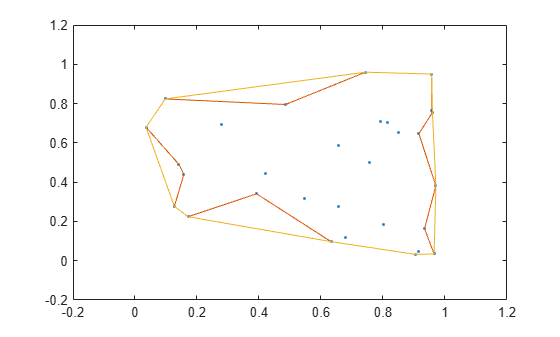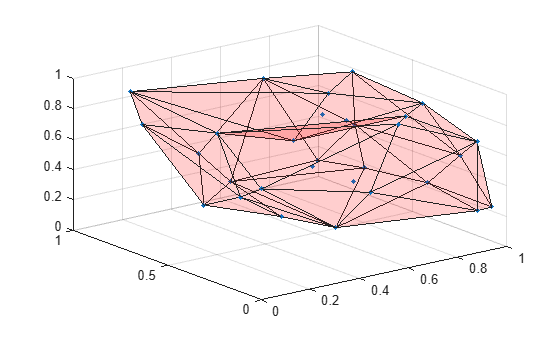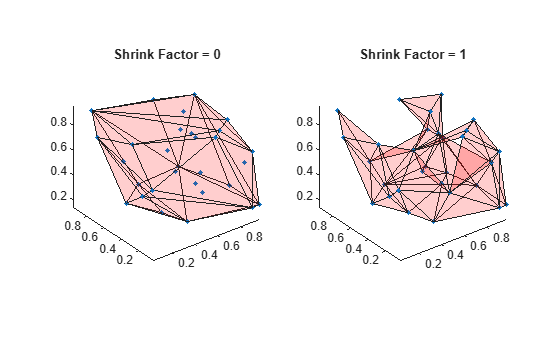# boundary

Boundary of a set of points in 2-D or 3-D

## Syntax

``k = boundary(x,y)``
``k = boundary(x,y,z)``
``k = boundary(P)``
``k = boundary(___,s)``
``````[k,v] = boundary(___)``````

## Description

example

````k = boundary(x,y)` returns a vector of point indices representing a single conforming 2-D boundary around the points `(x,y)`. The points `(x(k),y(k))` form the boundary. Unlike the convex hull, the boundary can shrink towards the interior of the hull to envelop the points.```

example

````k = boundary(x,y,z)` returns a triangulation representing a single conforming 3-D boundary around the points `(x,y,z)`. Each row of `k` is a triangle defined in terms of the point indices.```

example

````k = boundary(P)` specifies points `(x,y)` or `(x,y,z)` in the columns of matrix `P`.```

example

````k = boundary(___,s)` specifies shrink factor `s` using any of the previous syntaxes. `s` is a scalar between `0` and `1`. Setting `s` to `0` gives the convex hull, and setting `s` to `1` gives a compact boundary that envelops the points. The default shrink factor is `0.5`.```

example

``````[k,v] = boundary(___)``` also returns a scalar `v`, which is the area (2-D) or volume (3-D) which boundary `k` encloses.```

## Examples

collapse all

Create and plot a set of random 2-D points.

```rng('default') x = rand(30,1); y = rand(30,1); plot(x,y,'.') xlim([-0.2 1.2]) ylim([-0.2 1.2])```Compute a boundary around the points using the default shrink factor.

```k = boundary(x,y); hold on; plot(x(k),y(k));```Create a new boundary around the points using a shrink factor of 0.1. The result is a less compact boundary enveloping the points.

```j = boundary(x,y,0.1); hold on; plot(x(j),y(j));```Create and plot a set of random 3-D points.

```rng('default') P = rand(30,3); plot3(P(:,1),P(:,2),P(:,3),'.','MarkerSize',10) grid on```Plot the boundary using the default shrink factor.

```k = boundary(P); hold on trisurf(k,P(:,1),P(:,2),P(:,3),'Facecolor','red','FaceAlpha',0.1)```Create and plot a set of random 3-D points.

```rng default; P = rand(30,3); plot3(P(:,1),P(:,2),P(:,3),'.') grid on```Compute two boundaries: one with a shrink factor of 0 and the other with a shrink factor of 1.

```k = boundary(P,0); j = boundary(P,1);```

Compare the shrink factors by plotting the original points and the two boundaries side-by-side.

```subplot(1,2,1); plot3(P(:,1),P(:,2),P(:,3),'.','MarkerSize',10) hold on trisurf(k,P(:,1),P(:,2),P(:,3),'FaceColor','red','FaceAlpha',0.1) axis equal title('Shrink Factor = 0') subplot(1,2,2); plot3(P(:,1),P(:,2),P(:,3),'.','MarkerSize',10) hold on trisurf(j,P(:,1),P(:,2),P(:,3),'FaceColor','red','FaceAlpha',0.1) axis equal title('Shrink Factor = 1')```Create and plot a set of random 3-D points.

```rng('default') P = rand(30,3); plot3(P(:,1),P(:,2),P(:,3),'.') grid on```Use the `boundary` function to compute a boundary around the points, and find the volume of the resulting shape.

```[~, vol] = boundary(P); vol```
```vol = 0.2962 ```

## Input Arguments

collapse all

x-coordinates of points, specified as a column vector.

Data Types: `double`

y-coordinates of points, specified as a column vector.

Data Types: `double`

z-coordinates of points, specified as a column vector.

Data Types: `double`

Point coordinates, specified as a matrix with two columns (for a 2-D alpha shape) or a matrix with three columns (for a 3-D alpha shape).

• For 2-D, the columns of `P` represent `x` and `y` coordinates, respectively.

• For 3-D, the columns of `P` represent `x`, `y`, and `z` coordinates, respectively.

Data Types: `double`

Shrink factor, specified as a scalar in the range of `[0,1]`.

• `s = 0` corresponds to the convex hull of the points.

• `s = 1` corresponds to the tightest single-region boundary around the points.

The default shrink factor is `0.5`. Specify a larger or smaller shrink factor to tighten or loosen the boundary around the points, respectively.

Example: `k = boundary(x,y,0.76)` specifies a shrink factor of `0.76`, producing a tighter boundary than the default.

## Output Arguments

collapse all

Boundary point indices, returned as a vector or matrix. `k` contains the indices of the input points that lie on the boundary.

• For 2-D problems, `k` is a column vector of point indices representing the sequence of points around the boundary, which is a polygon.

• For 3-D problems, `k` is a triangulation matrix of size `mtri`-by-`3`, where `mtri` is the number of triangular facets on the boundary. Each row of `k` defines a triangle in terms of the point indices, and the triangles collectively form a bounding polyhedron.

Area or volume enclosed by boundary, returned as a scalar.

• For 2-D problems, `v` is the area enclosed by boundary `k`.

• For 3-D problems, `v` is the volume enclosed by boundary `k`.

## Algorithms

`boundary` constructs an `alphaShape` from the specified points and then uses `boundaryFacets` to determine which points lie on the boundary.

## Version History

Introduced in R2014b